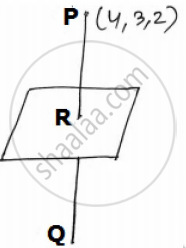# Find the Coordinates of the Foot of Perpendicular and Perpendicular Distance from the Point P(4,3,2) to the Plane X + 2y + 3z = 2. Also Find the Image of P in the Plane. - Mathematics

Sum

Find the coordinates of the foot of perpendicular and perpendicular distance from the point P(4,3,2) to the plane x + 2y + 3z = 2. Also find the image of P in the plane.

#### Solution

Let pi : "x" + 2"y" + 3"z" = 2.

The d.r.'s of its normal are 1, 2, 3.

Draw PR ⊥ plane pi.

So, equation of PR : ("x"-4)/(1) = ("y"-3)/(2) = ("z"-2)/(3) = λ  "say"

Coordinates of any random point on the line PR is,
R(λ+ 4,2λ  +3λ  +2).

As R lies on the plane so,
λ + 4 +2(2λ  + 3) + 3(3λ  + 3) = 2
⇒ -1.

So, foot of perpendicular is R(3. 1, -1).

And, perpendicular distance, PR = sqrt((3-4)^2 + (1-3)^2 + (-1-2)^2)

∴ PR = sqrt14  "units".

Let Q(h, p,s) be the image of point P in the plane.So, R must be the mid-point of PQ.

That is, "R" (("h"+4)/(2),("p"+3)/(2),("s"+2)/(2)) = "R"(3,1,-1)

On comparing the coordinates, ("h"+4)/(2)=3,("p"+3)/(2)=1,("s"+2)/(2)=-1

⇒ "h" = 2, "p" =-1, "s"=-4

Hence the image is Q(2, -1, -4).

Perpendicular distance PR = sqrt14`

Concept: Three - Dimensional Geometry Examples and Solutions
Is there an error in this question or solution?